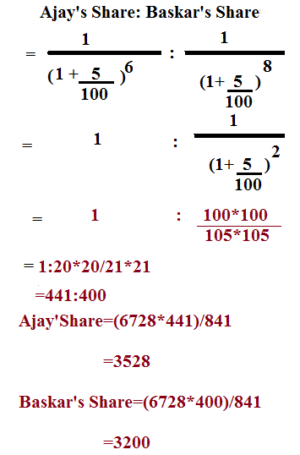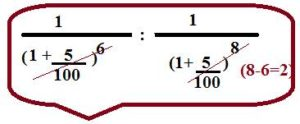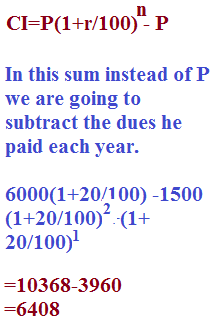# DIFFICULT PROBLEMS ON SIMPLE INTEREST & COMPOUND INTEREST-HOW TO SERIES

Hi Bankersdaily Aspirants,

Learning is a step by step process and you should gradually progress in that to ace the process. As you all know we have started a new Learn Series to help with your preparations for various exams like IBPS PO , IBPS RRB Scale I officer , IBPS RRB Office Assistant , IBPS Clerk , IBPS SO , SBI PO , SBI Clerk , SBI SO and many other exams in this type. Nevertheless this series will also contribute you all the tips and tricks to every miscellaneous sections and also all the important topics which are asked in the exams.Before that let us take a look on Simple Interest & Compound Interest.

TOPICS TO BE DISCUSSED

1)BASED ON DIVIDING SUM FOR 2 DIFFERENT RATES

2)BASED ON CLEARING ALL HIS DUES

3)BASED ON DIFFERENT AMOUNT.

## #.1 TYPE 1:

### BASED ON DIVIDING SUM FOR 2 DIFFERENT RATES:

1)Divide the sum of Rs.6728 between Ajay & Baskar.So that the Shares of Ajay at the end of 6 years may be equal to the Shares of Baskar at the end of 8 years,Compound interest being 5%?

EXPLANATION

Whenever the Total Amount is given & their rate & number of years given,it is easy to calculate,### WILL YOU SOLVE THIS SUM:

1)Divide the Amount 11272 between Anil & Aneesh,In way that Anil’s share at the end of 10 year is equal to Aneesh Share at the end of 12 years,compound interest being at 12%?

Their Ratio’s are 784:625

The Share of Anil=6232

The Share of Aneesh=5000

2)Divide the Amount 20310 between Meenesh & Vinesh ,In a manner that Meenesh’s Share at the end of 5 years is equal to Vinesh Share at the end of 7 years,compound interest being at 8%?

Their Ratio is = 729:625

The Share of Meenesh=10935

The Share of Vinesh=9375

## #.2 TYPE 2:

### BASED ON CLEARING ALL HIS DUES:

1)A Man Borrows Rs.6000 at 20 % Compound rate of interest.At the end of each year he pays back Rs.1500.How much amount should he pay at the end of the third year to clear all his dues?

EXPLANATION### PRACTICE THIS SUM:

Thakur borrows Rs.8000 at 10% compound rate of interest.At the end of each year he pays back Rs.2000.How much amount should he pay at the end of the third year to clear all his dues?

He should Pay Rs.6028 at the end

2)Anil borrows Rs.1500 at 5% compound rate of interest.At the end of each year he pays back Rs.500.How much amount should he pay at the end of the third year to clear all his dues?

Rs.660 3/16

## #.3.TYPE 3:

### BASED ON DIFFERENT AMOUNT:

1)An Amount of money grows upto Rs.5100 in 2 years & upto Rs.5800 in 3 years on compound interest.Find the rate percent?

EXPLANATION

There is a simple method to solve these kind problems

### Rate=Difference between the amount/Amount in N year

Rate=700*100/5100

Rate=12.5%

Remember this Formula is applicable only if the difference between the year is 1 or the given year should be continuous

### PRACTICE THIS SUM:

1)An Amount of money grows upto Rs.13000 in 9 years & upto Rs.13780 in 10 years on compound interest.Find the rate percent?

Difference = 780

Therefore,780*100/13000=6%

2)An Amount of money grows upto Rs.18000 in 24 years & upto Rs.19440 in 25 years on compound interest.Find the rate percent?